# 14. Mathematics for Orbits: Ellipses, Parabolas, Hyperbolas

Michael Fowler

## Preliminaries: Conic Sections

Ellipses, parabolas and hyperbolas can all be generated by cutting a cone with a plane (see diagrams, from Wikimedia Commons). Taking the cone to be ${x}^{2}+{y}^{2}={z}^{2},$ and substituting the $z$ in that equation from the planar equation $\stackrel{\to }{r}\cdot \stackrel{\to }{p}=p,$ where $\stackrel{\to }{p}$ is the vector perpendicular to the plane from the origin to the plane,  gives a quadratic equation in $x,y.$ This translates into a quadratic equation in the plane$—$take the line of intersection of the cutting plane with the $x,y$ plane as the $y$ axis in both, then one is related to the other by a scaling ${x}^{\prime }=\lambda x.$  To identify the conic, diagonalized the form, and look at the coefficients of ${x}^{2},{y}^{2}.$ If they are the same sign, it is an ellipse, opposite, a hyperbola.  The parabola is the exceptional case where one is zero, the other equates to a linear term.

It is instructive to see how an important property of the ellipse follows immediately from this construction.The slanting plane in the figure cuts the cone in an ellipse. Two spheres inside the cone, having circles of contact with the cone $C,{C}^{\prime }$, are adjusted in size so that they both just touch the plane, at points $F,{F}^{\prime }$ respectively.

It is easy to see that such spheres exist, for example start with a tiny sphere inside the cone near the point, and gradually inflate it, keeping it spherical and touching the cone, until it touches the plane. Now consider a point $P$ on the ellipse. Draw two lines: one from $P$ to the point $F$ where the small sphere touches, the other up the cone, aiming for the vertex, but stopping at the point of intersection with the circle $C.$  Both these lines are tangents to the small sphere, and so have the same length. (The tangents to a sphere from a point outside it form a cone, they are all of equal length.)  Now repeat with ${F}^{\prime },{C}^{\prime }.$ We find that $PF+P{F}^{\prime }=PC+P{C}^{\prime },$ the distances to the circles measured along the line through the vertex.  So $PF+P{F}^{\prime }=C{C}^{\prime }$ in the obvious notation$—$ $F,{F}^{\prime }$ are therefore evidently the foci of the ellipse.

## The Ellipse

### Squashed Circles and Gardeners

The simplest nontrivial planetary orbit is a circle: ${x}^{2}+{y}^{2}={a}^{2}$ is centered at the origin and has radius $a.$  An ellipse is a circle scaled (squashed) in one direction, so an ellipse centered at the origin with semimajor axis $a$ and semiminor axis $b has equation

$\frac{{x}^{2}}{{a}^{2}}+\frac{{y}^{2}}{{b}^{2}}=1$

in the standard notation, a circle of radius $a$ scaled by a factor $b/a$ in the $y$ direction. (It’s usual to orient the larger axis along $x.$ )

A circle can also be defined as the set of points which are the same distance $a$ from a given point, and an ellipse can be defined as the set of points such that the sum of the distances from two fixed points is a constant length (which must obviously be greater than the distance between the two points!).  This is sometimes called the gardener’s definition: to set the outline of an elliptic flower bed in a lawn, a gardener would drive in two stakes, tie a loose string between them, then pull the string tight in all different directions to form the outline.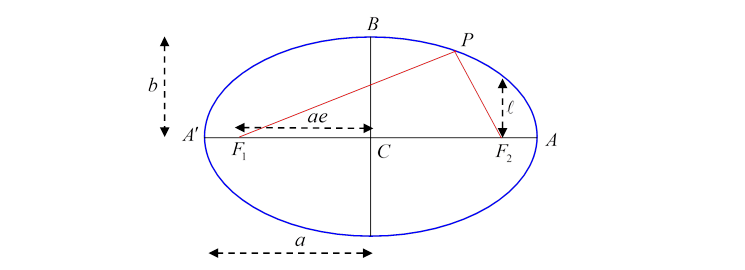In the diagram, the stakes are at ${F}_{1},{F}_{2}$, the red lines are the string, $P$ is an arbitrary point on the ellipse.

$CA$ is called the semimajor axis length $a$, $CB$  the semiminor axis, length $b$

${F}_{1},{F}_{2}$ are called the foci (plural of focus).

Notice first that the string has to be of length $2a$, because it must stretch along the major axis from ${F}_{1}$  to $A$ then back to ${F}_{2}$, and for that configuration there’s a double length of string along ${F}_{2}A$ and a single length from ${F}_{1}$ to ${F}_{2}$.  But the length ${A}^{\prime }{F}_{1}$ is the same as ${F}_{2}A$, so the total length of string is the same as the total length  ${A}^{\prime }A=2a.$

Suppose now we put $P$ at $B$.  Since ${F}_{1}B=B{F}_{2},$ and the string has length $2a$, the length ${F}_{1}B=a.$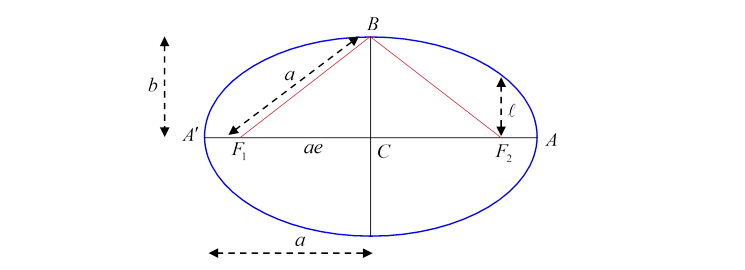We get a useful result by applying Pythagoras’ theorem to the triangle ${F}_{1}BC,$

${\left({F}_{1}C\right)}^{2}={a}^{2}-{b}^{2}.$

(We shall use this shortly.)

Evidently, for a circle, ${F}_{1}C=0.$

### Eccentricity

The eccentricity $e$ of the ellipse is defined by

Eccentric just means off center, this is how far the focus is off the center of the ellipse, as a fraction of the semimajor axis. The eccentricity of a circle is zero. The eccentricity of a long thin ellipse is just below one.

${F}_{1}$ and ${F}_{2}$ on the diagram are called the foci of the ellipse (plural of focus) because if a point source of light is placed at ${F}_{1}$, and the ellipse is a mirror, it will reflect$—$and therefore focus$—$all the light to ${F}_{2}$.

### Equivalence of the Two Definitions

We need to verify, of course, that this gardener’s definition of the ellipse is equivalent to the squashed circle definition.  From the diagram, the total string length

$2a={F}_{1}P+P{F}_{2}=\sqrt{{\left(x+ae\right)}^{2}+{y}^{2}}+\sqrt{{\left(x-ae\right)}^{2}+{y}^{2}}$

and squaring both sides of

$2a-\sqrt{{\left(x+ae\right)}^{2}+{y}^{2}}=\sqrt{{\left(x-ae\right)}^{2}+{y}^{2}}$

then rearranging to have the residual square root by itself on the left-hand side, then squaring again,

${\left(x+ae\right)}^{2}+{y}^{2}={\left(a+ex\right)}^{2}$,

from which, using ${e}^{2}=1-\left({b}^{2}/{a}^{2}\right),$ we find ${x}^{2}/{a}^{2}+{y}^{2}/{b}^{2}=1.$

### Ellipse in Polar Coordinates

In fact, in analyzing planetary motion, it is more natural to take the origin of coordinates at the center of the Sun rather than the center of the elliptical orbit.

It is also more convenient to take $\left(r,\theta \right)$ coordinates instead of $\left(x,y\right)$ coordinates, because the strength of the gravitational force depends only on $r$.  Therefore, the relevant equation describing a planetary orbit is the $\left(r,\theta \right)$ equation with the origin at one focus, here we follow the standard usage and choose the origin at ${F}_{2}.$

For an ellipse of semi major axis $a$  and eccentricity $e$  the equation is:

$\frac{a\left(1-{e}^{2}\right)}{r}=1+e\mathrm{cos}\theta .$

This is also often written

$\frac{\ell }{r}=1+e\mathrm{cos}\theta$

where $\ell$ is the semi-latus rectum, the perpendicular distance from a focus to the curve (so $\theta =\pi /2$ ), see the diagram below: but notice again that this equation has ${F}_{2}$ as its origin! (For $\theta <\pi /2,\text{ }r<\ell$.)

(It’s easy to prove $\ell =a\left(1-{e}^{2}\right)$ using Pythagoras’ theorem, ${\left(2a-\ell \right)}^{2}={\left(2ae\right)}^{2}+{\ell }^{2}$.)

The directrix: writing $r\mathrm{cos}\theta =x,$ the equation for the ellipse can also be written as

$r=a\left(1-{e}^{2}\right)-ex=e\left({x}_{0}-x\right)$,

where ${x}_{0}=\left(a/e\right)-ae$ (the origin $x=0$ being the focus).

The line $x={x}_{0}$ is called the directrix.

For any point on the ellipse, its distance from the focus is $e$ times its distance from the directrix.

### Deriving the Polar Equation from the Cartesian Equation

Note first that (following standard practice) coordinates $\left(x,y\right)$ and $\left(r,\theta \right)$ have different origins!

Writing $x=ae+r\mathrm{cos}\theta ,\text{\hspace{0.17em}}\text{\hspace{0.17em}}y=r\mathrm{sin}\theta$ in the Cartesian equation,

$\frac{{\left(ae+r\mathrm{cos}\theta \right)}^{2}}{{a}^{2}}+\frac{{\left(r\mathrm{sin}\theta \right)}^{2}}{{b}^{2}}=1,$

that is, with slight rearrangement,

${r}^{2}\left(\frac{{\mathrm{cos}}^{2}\theta }{{a}^{2}}+\frac{{\mathrm{sin}}^{2}\theta }{{b}^{2}}\right)+r\frac{2e\mathrm{cos}\theta }{a}-\left(1-{e}^{2}\right)=0.$

This is a quadratic equation for $r$ and can be solved in the usual fashion, but looking at the coefficients, it’s evidently a little easier to solve the corresponding quadratic for $u=1/r.$

The solution is:

$\frac{1}{r}=u=\frac{e\mathrm{cos}\theta }{a\left(1-{e}^{2}\right)}±\frac{1}{a\left(1-{e}^{2}\right)},$

from which

$\frac{a\left(1-{e}^{2}\right)}{r}=\frac{\ell }{r}=1+e\mathrm{cos}\theta$

where we drop the other root because it gives negative $r,$ for example for $\theta =\pi /2.$ This establishes the equivalence of the two equations.

## The Parabola

The parabola can be defines as the limiting curve of an ellipse as one focus (in the case we’re examining, that would be ${F}_{1}$ ) going to infinity.  The eccentricity evidently goes to one, $e\to 1$, since the center of the ellipse has gone to infinity as well. The semi-latus rectum $\ell$ is still defined as the perpendicular distance from the focus to the curve, the equation is

$\ell =r\left(1+\mathrm{cos}\theta \right).$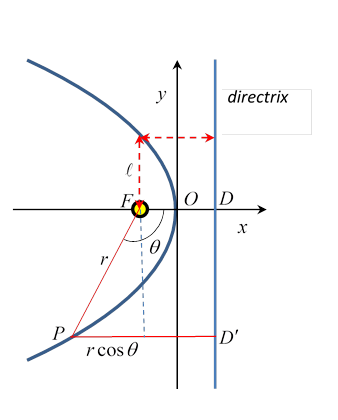Note that this describes a parabola opening to the left.  Taking $OF=1,$ the equation of this parabola is

${y}^{2}=-4x.$

All parabolas look the same, apart from scaling (maybe just in one direction).

The line perpendicular to the axis and the same distance from the curve along the axis as the focus is, but outside the curve, is the parabola’s directrix. That is, $FO=OD.$

Each point on the curve is the same distance from the focus as it is from the directrix.  This can be deduced from the limit of the ellipse property that the sum of distances to the two foci is constant.  Let’s call the other focus $\infty$. Then $FP+P\infty =FO+O\infty =D\infty ={D}^{\prime }\infty .$ So from the diagram, $FP=P{D}^{\prime }.$

Exercises: 1. Prove by finding the slope, etc., that any ray of light emitted by a point lamp at the focus will be reflected by a parabolic mirror to go out parallel to the axis.

2.  From the diagram above, show that the equality $FP=P{D}^{\prime }$ easily gives the equation for the parabola, both in $\left(r,\theta \right)$ and in $\left(x,y\right)$ coordinates.

## The Hyperbola

### Cartesian Coordinates

The hyperbola has eccentricity $e>1.$ In Cartesian coordinates, it has equation

$\frac{{x}^{2}}{{a}^{2}}-\frac{{y}^{2}}{{b}^{2}}=1$

and has two branches, both going to infinity approaching asymptotes $x=±\left(a/b\right)y.$ The curve intersects the $x$ axis at $x=±a$, the foci are at $x=±ae$, for any point on the curve,

${r}_{{F}_{1}}-{r}_{{F}_{2}}=±\text{\hspace{0.17em}}2a$

the sign being opposite for the two branches.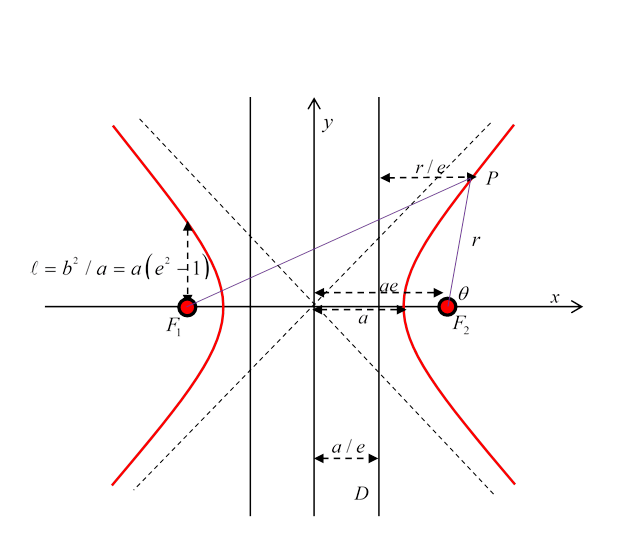The semi-latus rectum, as for the earlier conics, is the perpendicular distance from a focus to the curve, and is $\ell ={b}^{2}/a=a\left({e}^{2}-1\right).$ Each focus has an associated directrix, the distance of a point on the curve from the directrix multiplied by the eccentricity gives its distance from the focus.

### Polar Coordinates

The $\left(r,\theta \right)$ equation with respect to a focus can be found by substituting $x=r\mathrm{cos}\theta +ae,$ $y=r\mathrm{sin}\theta$ in the Cartesian equation and solving the quadratic for $u=1/r.$

Notice that $\theta$ has a limited range: the equation for the right-hand curve with respect to its own focus ${F}_{2}$ has

$\mathrm{tan}{\theta }_{\text{asymptote}}=±b/a$, so $\mathrm{cos}{\theta }_{\text{asymptote}}=±1/e.$

The equation for this curve is

$\frac{\ell }{r}=1-e\mathrm{cos}\theta$

in the range

${\theta }_{\text{asymptote}}<\theta <2\pi -{\theta }_{\text{asymptote}}.$

This equation comes up with various signs!  The left hand curve, with respect to the left hand focus, would have a positive sign $+e.$   With origin at ${F}_{1},$ (on the left) the equation of the right-hand curve is $\frac{\ell }{r}=e\mathrm{cos}\theta -1,$ finally with the origin at ${F}_{2}$ the left-hand curve is $\frac{\ell }{r}=-1-e\mathrm{cos}\theta .$ These last two describe repulsive inverse square scattering (Rutherford).

### Note:  A Useful Result for Rutherford Scattering

If we define the hyperbola by

$\frac{{x}^{2}}{{a}^{2}}-\frac{{y}^{2}}{{b}^{2}}=1,$

then the perpendicular distance from a focus to an asymptote is just $b.$

This equation is the same (including scale) as

$\ell /r=-1-e\mathrm{cos}\theta$, with $\ell ={b}^{2}/a=a\left({e}^{2}-1\right).$

#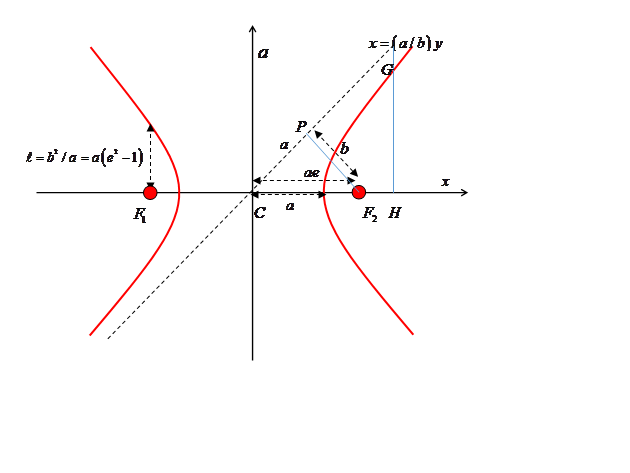Proof:  The triangle $CP{F}_{2}$ is similar to triangle $CHG$, so $P{F}_{2}/PC=GH/CH=b/a$, and since the square of the hypotenuse $C{F}_{2}$  is ${a}^{2}{e}^{2}={a}^{2}+{b}^{2}$, the distance ${F}_{2}P=b.$

I find this a surprising result because in analyzing Rutherford scattering (and other scattering) the impact parameter, the distance of the ingoing particle path from a parallel line through the scattering center, is denoted by $b.$  Surely this can’t be a coincidence? But I can’t find anywhere that this was the original motivation for the notation.